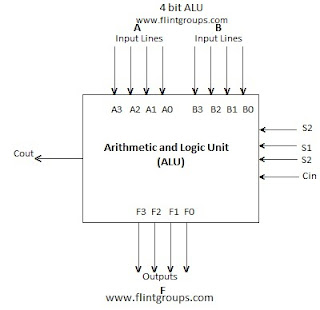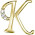# Arithmetic Logic Unit (ALU)

### Arithmetic Logic Unit

Arithmetic Logic Unit(ALU) is a Multioperation Combinational logic circuit that can perform various arithmetic and logicl operations.It is the heart of any microprocessor/microcontroller system.

The block diagram of a 4 bit ALU is shown below.The basic arithmetic operation performed by a ALU are Addition ,Subtraction, Multiplication, Division  and etc. The Basic Logical Operations Performed by ALU are AND,OR,EX-OR,NOT etc.
A and B are two four bit input lines which is used to select a particular operation in the unit.The selection lines are decoded within the ALU.F is the output.S2 is mode select.It is set to 0 for Arithmetic Operation and 1 for logical Operations S1 and S0 are select lines used for performing four types of Arithmetic or Logical Operations.Cin and Cout are carry input and output lines.

Functional Table#### 1 comment:

1.Great Article. i read every post in this blog.

Find Daily GK Updates, Government Jobs, bank recruitment.